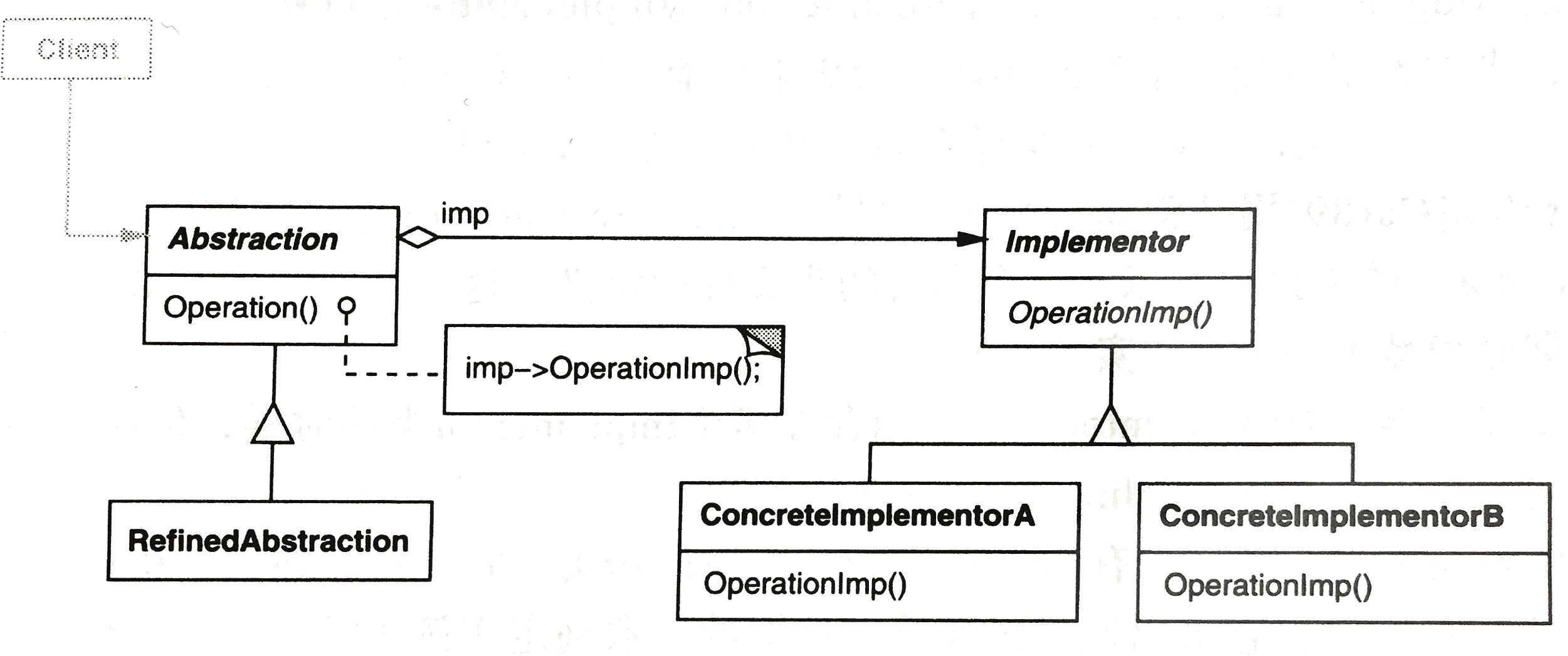设计模式：桥接模式|菜鸟教程

介绍1. 猪八戒从天蓬元帅转世投胎到猪，转世投胎的机制将尘世划分为两个等级，即：灵魂和肉体，前者相当于抽象化，后者相当于实现化。生灵通过功能的委派，调用肉体对象的功能，使得生灵可以动态地选择。
2. 墙上的开关，可以看到的开关是抽象的，不用管里面具体怎么实现的。

1. 抽象和实现的分离。
2. 优秀的扩展能力。
3. 实现细节对客户透明。

1. 如果一个系统需要在构件的抽象化角色和具体化角色之间增加更多的灵活性，避免在两个层次之间建立静态的继承联系，通过桥接模式可以使它们在抽象层建立一个关联关系。
2. 对于那些不希望使用继承或因为多层次继承导致系统类的个数急剧增加的系统，桥接模式尤为适用。
3. 一个类存在两个独立变化的维度，且这两个维度都需要进行扩展。

1. 首先，在形式上，两者还是有一定区别的，对比两幅结构图，我们可以发现，在桥接模式中不仅Implementor具有变化 （ConcreateImplementior），而且Abstraction也可以发生变化（RefinedAbstraction），而且两者的变化 是完全独立的，RefinedAbstraction与ConcreateImplementior之间松散耦合，它们仅仅通过Abstraction与 Implementor之间的关系联系起来。而在策略模式中，并不考虑Context的变化，只有算法的可替代性。

1. 其次在语意上，桥接模式强调Implementor接口仅提供基本操作，而Abstraction则基于这些基本操作定义更高层次的操作。而策略模式强调 Strategy抽象接口的提供的是一种算法，一般是无状态、无数据的，而Context则简单调用这些算法完成其操作。

2. 桥接模式中不仅定义Implementor的接口而且定义Abstraction的接口，Abstraction的接口不仅仅是为了与 Implementor通信而存在的，这也反映了结构型模式的特点：通过继承、聚合的方式组合类和对象以形成更大的结构。在策略模式中，Startegy 和Context的接口都是两者之间的协作接口，并不涉及到其它的功能接口，所以它是行为模式的一种。行为模式的主要特点就是处理的是对象之间的通信方 式，往往是通过引入中介者对象将通信双方解耦，在这里实际上就是将Context与实际的算法提供者解耦。

实现CPP 实现

#include <iostream>
#include <string>

using namespace std;

class DrawAPI
{
public:
virtual void drawCircle(int x, int y, int radius) = 0;
};

class Shape
{
public:
Shape(DrawAPI* drawAPI, int x, int y, int radius)
{
this->drawAPI = drawAPI;
this->x = x;
this->y = y;
}
virtual void draw() = 0;

protected:
DrawAPI *drawAPI;
int x;
int y;
};

#include "Interface.h"

class RedCircle:public DrawAPI
{
public:
void drawCircle(int x, int y, int radius);
};

class GreenCircle:public DrawAPI
{
public:
void drawCircle(int x, int y, int radius);
};

class Circle: public Shape
{
public:
Circle(int x, int y, int radius, DrawAPI* drawAPI);
void draw();
};

#include "Implement.h"

void RedCircle::drawCircle(int x, int y, int radius)
{
cout << "drawing circle[ color: red, radius: " << radius << ", x: " << x <<", "<< y <<"]" << endl;
}

void GreenCircle::drawCircle(int x, int y, int radius)
{
cout << "Drawing Circle[ color: green, radius: " << radius <<", x: " <<x<<", "<< y <<"]" << endl;
}

{
}

void Circle::draw()
{
}

#include "Implement.h"

int main(int argc, char** argv)
{
Shape* redCircle = new Circle(100, 100, 10, new RedCircle());
Shape* greenCircle = new Circle(100, 200, 10, new GreenCircle());

redCircle->draw();
greenCircle->draw();

return 0;
}

drawing circle[ color: red, radius: 10, x: 100, 100]
Drawing Circle[ color: green, radius: 10, x: 100, 200]

Java实现

DrawAPI.java
public interface DrawAPI {
public void drawCircle(int radius, int x, int y);
}

RedCircle.java

public class RedCircle implements DrawAPI {
@Override
public void drawCircle(int radius, int x, int y) {
System.out.println("Drawing Circle[ color: red, radius: "
+ radius +", x: " +x+", "+ y +"]");
}
}

GreenCircle.java

public class GreenCircle implements DrawAPI {
@Override
public void drawCircle(int radius, int x, int y) {
System.out.println("Drawing Circle[ color: green, radius: "
+ radius +", x: " +x+", "+ y +"]");
}
}

Shape.java

public abstract class Shape {
protected DrawAPI drawAPI;
protected Shape(DrawAPI drawAPI){
this.drawAPI = drawAPI;
}
public abstract void draw();
}

Circle.java

public class Circle extends Shape {

public Circle(int x, int y, int radius, DrawAPI drawAPI) {
super(drawAPI);
this.x = x;
this.y = y;
}

public void draw() {
}
}

BridgePatternDemo.java

public class BridgePatternDemo {
public static void main(String[] args) {
Shape redCircle = new Circle(100,100, 10, new RedCircle());
Shape greenCircle = new Circle(100,100, 10, new GreenCircle());

redCircle.draw();
greenCircle.draw();
}
}

Drawing Circle[ color: red, radius: 10, x: 100, 100]
Drawing Circle[ color: green, radius: 10, x: 100, 100]